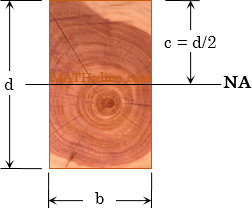# Bending Stress and Shearing Stress in Timber Beam

 Bending Stress $f_b = \dfrac{M}{S} = \dfrac{Mc}{I}$   Horizontal Shear Stress $f_v = \dfrac{VQ}{Ib}$For Rectangular Sections $f_b = \dfrac{6M}{bd^2}$   $f_v = \dfrac{3V}{2bd}$For Circular Sections $f_b = \dfrac{4M}{\pi r^3} = \dfrac{32M}{\pi d^3}$   $f_v = \dfrac{4V}{3\pi r^2} = \dfrac{16V}{3\pi d^2}$Where
fb = actual bending or flexural stress
fv = actual horizontal shear stress
M = maximum moment
V = maximum shear
c = distance from the neutral axis to the extreme fiber in bending
I = moment of inertial about the neutral axis
S = section modulus
Q = moment of area about the neutral axis